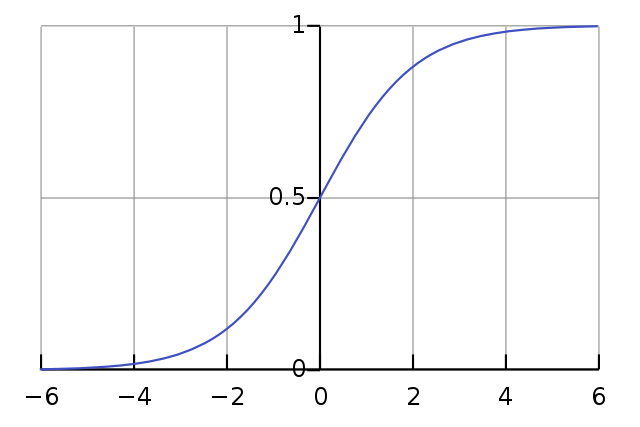# Help Generating an S-Curve/Sigmoid?

Hallo -
I am hoping to make a conventional “double s-curve”/sigmoid, like the attached image , but I’m finding it difficult to tune in correctly. Can anyone suggest an object to do this with?One way is to use Env:

``````Env([0, 1], , curve: 'sine').plot;
``````

Best,
Paul

Thank you! Is there a way to adjust the curvature on either end?

Yes using numbers for curve instead of sine. For s-shaped, 2 stages are needed:

``````Env([0, 0.5, 1], [1,1], curve: [-2, 2]).plot;
Env([0, 0.5, 1], [1,1], curve: [-4, 4]).plot;
Env([0, 0.5, 1], [1,1], curve: [-8, 8]).plot;
``````
1 Like

I know this marked as solved but… sometimes i use `.scurve` or `tanh` operator rather than going the `Env` route.

`(0,0s1..1).scurve.plot;`

Also works with .`hanWindow, welWindow.` etc.

I will see myself out.
Lukiss.

1 Like